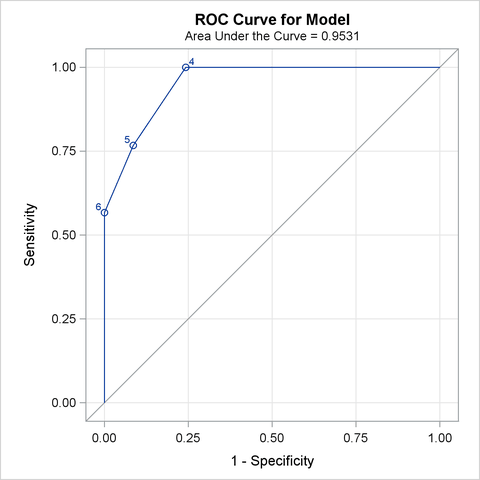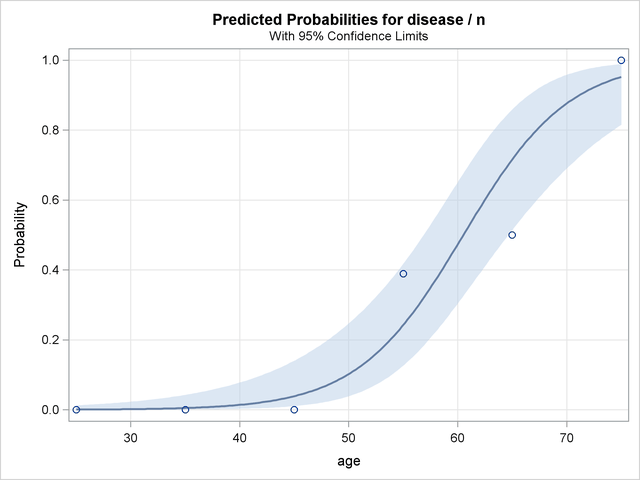## Example 53.7 ROC Curve, Customized Odds Ratios, Goodness-of-Fit Statistics, R-Square, and Confidence Limits

This example plots an ROC curve, estimates a customized odds ratio, produces the traditional goodness-of-fit analysis, displays the generalizedmeasures for the fitted model, calculates the normal confidence intervals for the regression parameters, and produces a display of the probability function and prediction curves for the fitted model. The data consist of three variables: n (number of subjects in the sample), disease (number of diseased subjects in the sample), and age (age for the sample). A linear logistic regression model is used to study the effect of age on the probability of contracting the disease. The statements to produce the data set and perform the analysis are as follows:

```data Data1;
input disease n age;
datalines;
0 14 25
0 20 35
0 19 45
7 18 55
6 12 65
17 17 75
;
```
```ods graphics on;
proc logistic data=Data1 plots(only)=roc(id=obs);
model disease/n=age / scale=none
clparm=wald
clodds=pl
rsquare;
units age=10;
effectplot;
run;
ods graphics off;
```

The option SCALE=NONE is specified to produce the deviance and Pearson goodness-of-fit analysis without adjusting for overdispersion. The RSQUARE option is specified to produce generalizedmeasures of the fitted model. The CLPARM=WALD option is specified to produce the Wald confidence intervals for the regression parameters. The UNITS statement is specified to produce customized odds ratio estimates for a change of 10 years in the age variable, and the CLODDS=PL option is specified to produce profile-likelihood confidence limits for the odds ratio. The PLOTS= option with ODS Graphics enabled produces a graphical display of the ROC curve, and the EFFECTPLOT statement displays the model fit.

The results in Output 53.7.1 show that the deviance and Pearson statistics indicate no lack of fit in the model.

Output 53.7.1 Deviance and Pearson Goodness-of-Fit Analysis
The LOGISTIC Procedure

Deviance and Pearson Goodness-of-Fit Statistics
Criterion Value DF Value/DF Pr > ChiSq
Deviance 7.7756 4 1.9439 0.1002
Pearson 6.6020 4 1.6505 0.1585

Number of events/trials observations: 6

Output 53.7.2 shows that the R-square for the model is 0.74. The odds of an event increases by a factor of 7.9 for each 10-year increase in age.

Output 53.7.2 R-Square, Confidence Intervals, and Customized Odds Ratio
Model Fit Statistics
Criterion Intercept
Only
Intercept
and
Covariates
With
Constant
AIC 124.173 52.468 18.075
SC 126.778 57.678 23.285
-2 Log L 122.173 48.468 14.075

 R-Square Max-rescaled R-Square 0.5215 0.7394

Testing Global Null Hypothesis: BETA=0
Test Chi-Square DF Pr > ChiSq
Likelihood Ratio 73.7048 1 <.0001
Score 55.3274 1 <.0001
Wald 23.3475 1 <.0001

Analysis of Maximum Likelihood Estimates
Parameter DF Estimate Standard
Error
Wald
Chi-Square
Pr > ChiSq
Intercept 1 -12.5016 2.5555 23.9317 <.0001
age 1 0.2066 0.0428 23.3475 <.0001

Association of Predicted Probabilities and
Observed Responses
Percent Concordant 92.6 Somers' D 0.906
Percent Discordant 2.0 Gamma 0.958
Percent Tied 5.4 Tau-a 0.384
Pairs 2100 c 0.953

Parameter Estimates and Wald Confidence
Intervals
Parameter Estimate 95% Confidence Limits
Intercept -12.5016 -17.5104 -7.4929
age 0.2066 0.1228 0.2904

Odds Ratio Estimates and Profile-Likelihood
Confidence Intervals
Effect Unit Estimate 95% Confidence Limits
age 10.0000 7.892 3.881 21.406

Since ODS Graphics is enabled, a graphical display of the ROC curve is produced as shown in Output 53.7.3.

Output 53.7.3 Receiver Operating Characteristic CurveNote that the area under the ROC curve is estimated by the statistic c in the "Association of Predicted Probabilities and Observed Responses" table. In this example, the area under the ROC curve is 0.953.

Since there is only one continuous covariate and since ODS Graphics is enabled, the EFFECTPLOT statement produces a graphical display of the predicted probability curve with bounding 95% confidence limits as shown in Output 53.7.4.

Output 53.7.4 Predicted Probability and 95% Prediction Limits